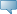## Catalog Entries

Fall 2020
Oct 01, 2022Select the link beside Class Schedule to find available classes for the course.

 MATH 2720 - Multivariable Calculus Calculus of several variables. For students in one of the following programs: Actuarial Mathematics, Data Science, Statistics (Honours or Majors), Physics (Honours or Majors) Geophysics (Honours or Majors), and Physical Geography. May not be held with the former MATH 2750, the former MATH 2110, MATH 2130, MATH 2150, MATH 2151 or MATH 2721. Prerequisites: (one of MATH 1220, MATH 1300, MATH 1301, or MATH 1310) and (one of MATH 1232, MATH 1690, MATH 1700, MATH 1701, MATH 1710, or the former MATH 1730). - 3.0 Credit hours Science Mathematics Department Course Attributes: Calculus, Mathematics Requirement, Science Requirement for BA, Science